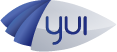#API Docs for: 3.8.0
Show:

# StackingUtil Class

Module: charts-base
Parent Module: charts

Utility class used for creating stacked series.

## Methods

### `_cleanXNaN`

(
• `xcoords`
• `ycoords`
)
private

Cleans invalid x-coordinates by calculating their value based on the corresponding y-coordinate, the previous valid x-coordinate with its corresponding y-coordinate and the next valid x-coordinate with its corresponding y-coordinate. If there is no previous or next valid x-coordinate, the value will not be altered.

#### Parameters:

• `xcoords` Array

An array of x-coordinate values

• `ycoords` Array

An arry of y-coordinate values

### `_cleanYNaN`

(
• `xcoords`
• `ycoords`
)
private

Cleans invalid y-coordinates by calculating their value based on the corresponding x-coordinate, the previous valid y-coordinate with its corresponding x-coordinate and the next valid y-coordinate with its corresponding x-coordinate. If there is no previous or next valid y-coordinate, the value will not be altered.

#### Parameters:

• `xcoords` Array

An array of x-coordinate values

• `ycoords` Array

An arry of y-coordinate values

### `_getNextValidCoordValue`

(
• `coords`
• `index`
)
private

Returns the next valid (numeric) value in an array if available.

#### Parameters:

• `coords` Array

Array of values

• `index` Number

The index in the array in which to begin searching.

Number

### `_getPreviousValidCoordValue`

(
• `coords`
• `index`
)
private

Returns the previous valid (numeric) value in an array if available.

#### Parameters:

• `coords` Array

Array of values

• `index` Number

The index in the array in which to begin searching.

Number

() protected

### `_stackXCoords`

() protected

Stacks coordinates for a stacked vertical series.

### `_stackYCoords`

() protected

Stacks coordinates for a stacked horizontal series.

## Properties

### `_stacked`

Unknown private

Indicates whether the series is stacked.